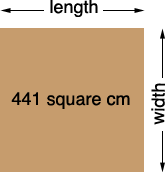SEARCH HOMEMath Central Quandaries & QueriesQuestion from tara, a parent: am trying to help my brother's daughter in maths, tomorrow she has maths exam 1. Area of sq. picture is 441 sq. cm what is the length of its side.Hi Tara,

Since the picture is a square its length and width are equal.Thus the area is

length × width= length × length = length2 = 441 square cm.

Thus length = √441 = 21 cm.

PennyMath Central is supported by the University of Regina and The Pacific Institute for the Mathematical Sciences.